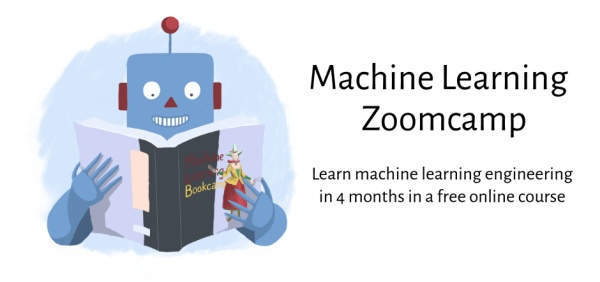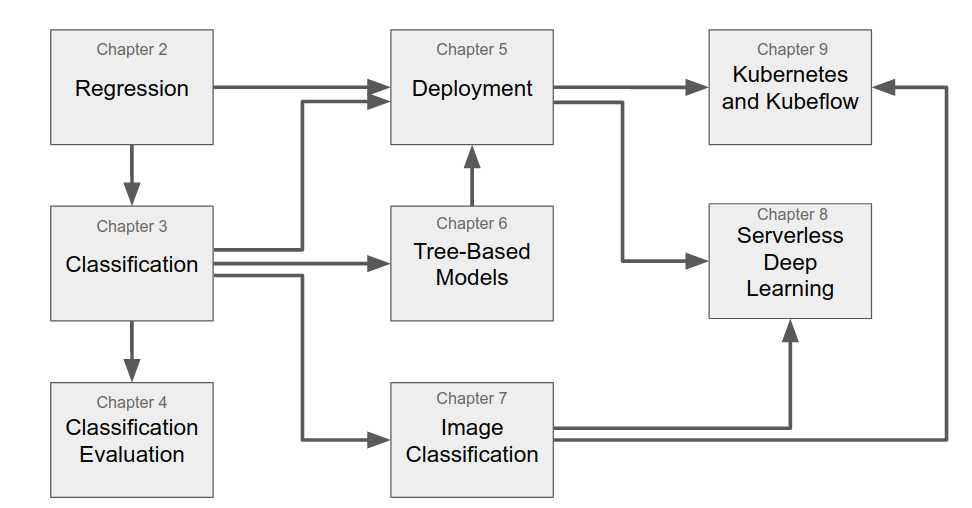# alexeygrigorev / mlbookcamp-code

The code from the Machine Learning Bookcamp book and a free course based on the book

# Machine Learning Bookcamp

The code from the Machine Learning Bookcamp book

## Machine Learning ZoomcampMachine Learning Zoomcamp is a course based on the book

• It's online and free
• You can join at any moment# Chapters

## Chapter 1: Introduction to Machine Learning

• Understanding machine learning and the problems it can solve
• CRISP-DM: Organizing a successful machine learning project
• Training and selecting machine learning models
• Performing model validation

No code

## Chapter 2: Machine Learning for Regression

• Creating a car-price prediction project with a linear regression model
• Doing an initial exploratory data analysis with Jupyter notebooks
• Setting up a validation framework
• Implementing the linear regression model from scratch
• Performing simple feature engineering for the model
• Keeping the model under control with regularization
• Using the model to predict car prices

## Chapter 3: Machine Learning for Classification

• Predicting customers who will churn with logistic regression
• Doing exploratory data analysis for identifying important features
• Encoding categorical variables to use them in machine learning models
• Using logistic regression for classification

## Chapter 4: Evaluation Metrics for Classification

• Accuracy as a way of evaluating binary classification models and its limitations
• Determining where our model makes mistakes using a confusion table
• Deriving other metrics like precision and recall from the confusion table
• Using ROC and AUC to further understand the performance of a binary classification model
• Cross-validating a model to make sure it behaves optimally
• Tuning the parameters of a model to achieve the best predictive performance

## Chapter 5: Deploying Machine Learning Models

• Saving models with Pickle
• Managing dependencies with Pipenv
• Making the service self-contained with Docker
• Deploying it to the cloud using AWS Elastic Beanstalk

## Chapter 6: Decision Trees and Ensemble Learning

• Predicting the risk of default with tree-based models
• Decision trees and the decision tree learning algorithm
• Random forest: putting multiple trees together into one model
• Gradient boosting as an alternative way of combining decision trees

## Chapter 7: Neural Networks and Deep Learning

• Convolutional neural networks for image classification
• TensorFlow and Keras — frameworks for building neural networks
• Using pre-trained neural networks
• Internals of a convolutional neural network
• Training a model with transfer learning
• Data augmentations — the process of generating more training data

## Chapter 8: Serverless Deep Learning

• Serving models with TensorFlow-Lite — a light-weight environment for applying TensorFlow models
• Deploying deep learning models with AWS Lambda
• Exposing the Lambda function as a web service via API Gateway

## Chapter 9: Kubernetes and Kubeflow

Kubernetes:

• Understanding different methods of deploying and serving models in the cloud.
• Serving Keras and TensorFlow models with TensorFlow-Serving
• Deploying TensorFlow-Serving to Kubernetes

Kubeflow:

• Using Kubeflow and KFServing for simplifying the deployment process

Code: chapter-09-kubeflow

Articles from mlbookcamp.com:

# Appendices

## Appendix A: Setting up the Environment

• Installing Anaconda, a Python distribution that includes most of the scientific libraries we need
• Running a Jupyter Notebook service from a remote machine
• Installing and configuring the Kaggle command line interface tool for accessing datasets from Kaggle
• Creating an EC2 machine on AWS using the web interface and the command-line interface

Code: no code

Articles from mlbookcamp.com:

## Appendix B: Introduction to Python

• Basic python syntax: variables and control-flow structures
• Collections: lists, tuples, sets, and dictionaries
• List comprehensions: a concise way of operating on collections
• Reusability: functions, classes and importing code
• Package management: using pip for installing libraries
• Running python scripts

Articles from mlbookcamp.com:

## Appendix C: Introduction to NumPy and Linear Algebra

• One-dimensional and two-dimensional NumPy arrays
• Generating NumPy arrays randomly
• Operations with NumPy arrays: element-wise operations, summarizing operations, sorting and filtering
• Multiplication in linear algebra: vector-vector, matrix-vector and matrix-matrix multiplications
• Finding the inverse of a matrix and solving the normal equation

Articles from mlbookcamp.com:

## Appendix C: Introduction to Pandas

• The main data structures in Pandas: DataFrame and Series
• Accessing rows and columns of a DataFrame
• Element-wise and summarizing operations
• Working with missing values
• Sorting and grouping

## Appendix D: AWS SageMaker

• Increasing the GPU quota limits
• Renting a Jupyter notebook with GPU in AWS SageMaker